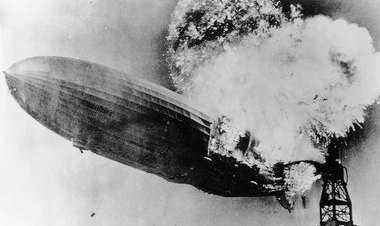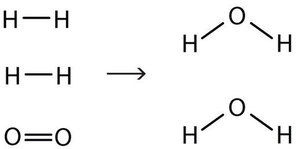# 6.2: Chemical Equations

$$\newcommand{\vecs}{\overset { \rightharpoonup} {\mathbf{#1}} }$$ $$\newcommand{\vecd}{\overset{-\!-\!\rightharpoonup}{\vphantom{a}\smash {#1}}}$$$$\newcommand{\id}{\mathrm{id}}$$ $$\newcommand{\Span}{\mathrm{span}}$$ $$\newcommand{\kernel}{\mathrm{null}\,}$$ $$\newcommand{\range}{\mathrm{range}\,}$$ $$\newcommand{\RealPart}{\mathrm{Re}}$$ $$\newcommand{\ImaginaryPart}{\mathrm{Im}}$$ $$\newcommand{\Argument}{\mathrm{Arg}}$$ $$\newcommand{\norm}{\| #1 \|}$$ $$\newcommand{\inner}{\langle #1, #2 \rangle}$$ $$\newcommand{\Span}{\mathrm{span}}$$ $$\newcommand{\id}{\mathrm{id}}$$ $$\newcommand{\Span}{\mathrm{span}}$$ $$\newcommand{\kernel}{\mathrm{null}\,}$$ $$\newcommand{\range}{\mathrm{range}\,}$$ $$\newcommand{\RealPart}{\mathrm{Re}}$$ $$\newcommand{\ImaginaryPart}{\mathrm{Im}}$$ $$\newcommand{\Argument}{\mathrm{Arg}}$$ $$\newcommand{\norm}{\| #1 \|}$$ $$\newcommand{\inner}{\langle #1, #2 \rangle}$$ $$\newcommand{\Span}{\mathrm{span}}$$$$\newcommand{\AA}{\unicode[.8,0]{x212B}}$$

Skills to Develop

• Define chemical reaction.
• Use a balanced chemical equation to represent a chemical reaction.

Water (H2O) is composed of hydrogen and oxygen. Suppose we imagine a process in which we take some elemental hydrogen (H2) and elemental oxygen (O2) and let them react to make water. The statement

"hydrogen and oxygen react to make water"

is one way to represent that process, which is called a chemical reaction. Figure $$\PageIndex{1}$$ shows a rather dramatic example of this very reaction.Figure $$\PageIndex{1}$$: The Formation of Water. Hydrogen and oxygen combine to form water. Here, the hydrogen gas in the zeppelin SS Hindenburg reacts with oxygen in the air to make water. Source: Photo courtesy of the US Navy. For a video of this see https://www.youtube.com/watch?v=CgWHbpMVQ1U.

To simplify the writing of reactions, we use formulas instead of names when we describe a reaction. We can also use symbols to represent other words in the reaction. A plus sign connects the initial substances (and final substances, if there is more than one), and an arrow (→) represents the chemical change:

$\ce{H_2 + O_2 \rightarrow H_2O} \label{Eq1}$

This statement is one example of a chemical equation, an abbreviated way of using symbols to represent a chemical change. The substances on the left side of the arrow are called reactants, and the substances on the right side of the arrow are called products. It is not uncommon to include a phase label with each formula—(s) for solid, (ℓ) for liquid, (g) for gas, and (aq) for a substance dissolved in water, also known as an aqueous solution. If we included phase labels for the reactants and products, under normal environmental conditions, the reaction would be as follows:

$\ce{H2(g) + O2(g) \rightarrow H2O (ℓ)} \label{Eq2}$

Chemical equations can also be used to describe physical changes. We will see examples of this soon.

This equation is still not complete because it does not satisfy the law of conservation of matter. Count the number of atoms of each element on each side of the arrow. On the reactant side, there are two H atoms and two O atoms; on the product side, there are two H atoms and only one oxygen atom. The equation is not balanced because the number of oxygen atoms on each side is not the same (Figure $$\PageIndex{2}$$).Figure $$\PageIndex{2}$$: Balanced—Yes or No?. By counting the atoms of each element, we can see that the reaction is not balanced as written.

To make this chemical equation conform to the law of conservation of matter, we must revise the amounts of the reactants and the products as necessary to get the same number of atoms of a given element on each side. Because every substance has a characteristic chemical formula, we cannot change the chemical formulas of the individual substances. For example, we cannot change the formula for elemental oxygen to O. However, we can assume that different numbers of reactant molecules or product molecules may be involved. For instance, perhaps two water molecules are produced, not just one:

$\ce{H2(g) + O2 (g) \rightarrow 2H2O (ℓ)} \label{Eq3}$

The 2 preceding the formula for water is called a coefficient. It implies that two water molecules are formed. There are now two oxygen atoms on each side of the equation.

This point is so important that we should repeat it. You cannot change the formula of a chemical substance to balance a chemical reaction! You must use the proper chemical formula of the substance.

Unfortunately, by inserting the coefficient 2 in front of the formula for water, we have also changed the number of hydrogen atoms on the product side as well. As a result, we no longer have the same number of hydrogen atoms on each side. This can be easily fixed, however, by putting a coefficient of 2 in front of the diatomic hydrogen reactant:

$\ce{2H2(g) + O2(g) \rightarrow 2H2O (ℓ)} \label{Eq4}$

Now we have four hydrogen atoms and two oxygen atoms on each side of the equation. The law of conservation of matter is satisfied because we now have the same number of atoms of each element in the reactants and in the products. We say that the reaction is balanced (Figure $$\PageIndex{3}$$). The diatomic oxygen has a coefficient of 1, which typically is not written but assumed in balanced chemical equations.Figure $$\PageIndex{3}$$: Balanced—Yes or No?. By counting the atoms of each element, we can see that the reaction is now balanced.

Proper chemical equations should be balanced. Writing balanced reactions is a chemist’s way of acknowledging the law of conservation of matter.

Example $$\PageIndex{1}$$

Is each chemical equation balanced?

1. 2Na(s) + O2(g) → 2Na2O(s)
2. CH4(g) + 2O2(g) → CO2(g) + 2H2O(ℓ)
3. AgNO3(aq) + 2KCl(aq) → AgCl(s) + KNO3(aq)

SOLUTION

1. By counting, we find two sodium atoms and two oxygen atoms in the reactants and four sodium atoms and two oxygen atoms in the products. This equation is not balanced.
2. The reactants have one carbon atom, four hydrogen atoms, and four oxygen atoms. The products have one carbon atom, four hydrogen atoms, and four oxygen atoms. This equation is balanced.
3. The reactants have one silver atom, one nitrogen atom, three oxygen atoms, two potassium atoms, and two chlorine atoms. The products have one silver atom, one chlorine atom, one potassium atom, one nitrogen atom, and three oxygen atoms. Because there are different numbers of chlorine and potassium atoms, this equation is not balanced.

Exercise $$\PageIndex{1}$$

Is each chemical equation balanced?

1. $$2Hg_{(ℓ)} + O_{2(g)} \rightarrow Hg_2O_{2(s)}$$
2. $$C_2H_{4(g)} + 2O_{2(g)} \rightarrow 2CO_{2(g)} + 2H2O_{(ℓ)}$$
3. $$Mg(NO_3)_{2(s)} + 2Li_{(s)} \rightarrow Mg_{(s)} + 2LiNO_{3(s)}$$

How does one balance a chemical equation, starting with the correct formulas of the reactants and products? Basically, a back-and-forth approach is adopted, counting the number of atoms of one element on one side, checking the number of atoms of that element on the other side, and changing a coefficient if necessary. Then check another element, going back and forth from one side of the equation to another, until each element has the same number of atoms on both sides of the arrow. In many cases, it does not matter which element is balanced first and which is balanced last, as long as all elements have the same number of atoms on each side of the equation.

For example, to balance the equation

$\ce{CH4 + Cl2 \rightarrow CCl4 + HCl} \label{Eq5}$

we might choose to count the carbon atoms first, finding that both sides are balanced with one carbon atom. The reactant side has four hydrogen atoms, so the product side must also have four hydrogen atoms. We fix this by putting a 4 in front of the HCl:

$\ce{CH4 + Cl2 \rightarrow CCl4 + 4HCl } \label{Eq6}$

Now each side has four hydrogen atoms. The product side has a total of eight chlorine atoms (four from the $$CCl_4$$ and four from the four molecules of HCl), so we need eight chlorine atoms as reactants. Because elemental chlorine is a diatomic molecule, we need four chlorine molecules to get a total of eight chlorine atoms. We add another 4 in front of the Cl2 reactant:

$\ce{CH4 + 4Cl2 \rightarrow CCl4 + 4HCl } \label{Eq7}$

Now we check: each side has one carbon atom, four hydrogen atoms, and eight chlorine atoms. The chemical equation is balanced.

Exercise $$\PageIndex{2}$$

1. What are the parts of a chemical equation?
2. Explain why chemical equations need to be balanced.

reactants and products

Chemical equations need to be balanced to satisfy the law of conservation of matter.

## Summary

Chemical reactions are represented by chemical equations that list reactants and products. Proper chemical equations are balanced; the same number of each element’s atoms appears on each side of the equation.

## Exercises

1. Write a chemical equation to express the fact that hydrogen gas and solid iodine react to make gaseous hydrogen iodide. Make sure the equation satisfies the law of conservation of matter.

2. Write a chemical equation to express the fact that sodium metal and chlorine gas react to make solid sodium chloride. Make sure the equation satisfies the law of conservation of matter.

3. Write an equation expressing the fact that hydrogen gas and fluorine gas react to make gaseous hydrogen fluoride. Make sure the equation satisfies the law of conservation of matter.

4. Write an equation expressing the fact that solid potassium and fluorine gas react to make solid potassium fluoride. Make sure the equation satisfies the law of conservation of matter.

5. Mercury reacts with oxygen to make mercury(II) oxide. Write a balanced chemical equation that summarizes this reaction.

6. Octane (C8H18) reacts with oxygen to make carbon dioxide and water. Write a balanced chemical equation that summarizes this reaction.

7. Propyl alcohol (C3H7OH) reacts with oxygen to make carbon dioxide and water. Write a balanced chemical equation that summarizes this reaction.

8. Sulfuric acid reacts with iron metal to make iron(III) sulfate and hydrogen gas. Write a balanced chemical equation that summarizes this reaction.

9. Balance each equation.

1. MgCl2 + K → KCl + Mg
2. C6H12O6 + O2 → CO2 + H2O
3. NaN3 → Na + N2 (This is the reaction used to inflate airbags in cars.)
10. Balance each equation.

1. NH4NO3 → N2O + H2O
2. TiBr4 + H2O → TiO2 + HBr
3. C3H5N3O9 → CO2 + N2 + O2 + H2O (This reaction represents the decomposition of nitroglycerine.)
11. Balance each equation.

1. NH3 + O2 → NO + H2O
2. Li + N2 → Li3N
3. AuCl → Au + AuCl3
12. Balance each equation.

1. NaOH + H3PO4 → Na3PO4 + H2O
2. N2H4 + Cl2 → N2 + HCl
3. Na2S + H2S → NaSH
13. Chromium(III) oxide reacts with carbon tetrachloride to make chromium(III) chloride and phosgene (COCl2). Write the balanced chemical equation for this reaction.

14. The reaction that occurs when an Alka-Seltzer tablet is dropped into a glass of water has sodium bicarbonate reacting with citric acid (H3C6H5O7) to make carbon dioxide, water, and sodium citrate (Na3C6H5O7). Write the balanced chemical equation for this reaction.

15. When sodium hydrogen carbonate is used to extinguish a kitchen fire, it decomposes into sodium carbonate, water, and carbon dioxide. Write a balanced chemical equation for this reaction.

16. Elemental bromine gas can be generated by reacting sodium bromide with elemental chlorine. The other product is sodium chloride. Write a balanced chemical equation for this reaction.

1. H2(g) + I2(s) → 2HI(g)

1. H2(g) + F2(g) → 2HF(g)

1. 2Hg + O2 → 2HgO

1. 2C3H7OH + 9O2 → 6CO2 + 8H2O

1. MgCl2 + 2K → 2KCl + Mg
2. C6H12O6 + 6O2 → 6CO2 + 6H2O
3. 2NaN3 → 2Na + 3N2
1. 4NH3 + 5O2 → 4NO + 6H2O
2. 6Li + N2 → 2Li3N
3. 3AuCl → 2Au + AuCl3
1. Cr2O3 + 3CCl4 → 2CrCl3 + 3COCl2

1. 2NaHCO3 → Na2CO3 + CO2 + H2O)

6.2: Chemical Equations is shared under a CC BY-NC-SA 4.0 license and was authored, remixed, and/or curated by LibreTexts.